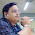### Man Ladder Bottle Puzzle - Knowledge Factory Puzzle

19 is the answer
Explanation
Equation 1: (7+3) + (7+3) + (7+3) = 30 (Man = 7 & Glass Bottle = 3 from equation 3)
Equation 2: 10 + 5 + 5 = 20 (Ladder = 5)
Equation 3: 5 + 3 + 3 = 11
Equation 4: 7 + (3 x 4) = 19 (Man = 7; Glass Bottle = 3 & Ladder = 4 as it has only 4 leg holders in last equation)

1.I don't understand last equation

2.forth equation will be - 7 + 3 * 5 = 22

1.Last 5 is wrong ladder legs are 4

3.The ladder in last line has 4 bars so value is 4
Rest all has 5 bars so value is 5

4.5.The answer is 25

6.The glass bottle showcase is exceptionally combined with local players commanding in their particular nations. 750ml empty glass bottles with screw caps